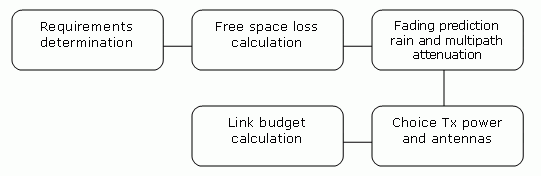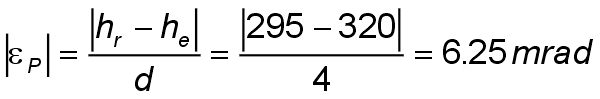# Example of microwave link design

Print version

## 2. Example of microwave link designFig. 2.1: Design flowchart

 Step 1 – Requirements Determination Link parameters: Link distance: 4 km First antenna height above sea level: 295 m Second antenna height above sea level: 320 m Location: Central Europe (rain zone H, refraction gradient dN1= −300) Transmission requirements: Required data rate: >160 Mbps Required availability: 99.99 % RAy parameters: 17 GHz Tx power +5 dBm (max. Tx power) Antenna gain: 30 cm    …  32.2 dBi 60 cm    …  37.8 dBi 99 cm    …  42 dBi Step 2 – Free space loss calculation FSL = 32.44 + 20log f + 20log D = 32.44 + 20log17.2·103 + 20log4 = 129.1 dB Step 3a – Rain attenuation For 99.99% availability in rain zone B the rain rate is R0.01=32 (see Fig. 1.1) For f=17 GHz kh=0.06146; αh=1.0949; kv=0.06797; αv=1.0137 Vertical polarization: Horizontal polarization: Step 3b – Attenuation due to multipath propagation We have to find required fade margin for reliability of the link 99.99 percent. Path inclination:The percentage of time that fade depth A (dB) is exceeded in the average worst month is calculated as: For reliability 99.99% is P0=0.01 we get exponential function for A: A = -0.19765 – 10log(0.01/0.022871) = 3.4 dB The minimum fade margin required to suppress multipath fading on this link would be 4 dB. Step 4 – Choice of Tx power and antennas Step 5 – and Link budget calculation Calculation in steps 3a and 3b determines the minimum fade margin required for stable link operation as 11 dB (rain attenuation is dominant). If you use the maximum performance of antenna with diameter of 30 cm, complete the radio formula as follows: PR = PT + GT + GR − FSL = 5 + 32.2 + 32.2 − 129.1 = −59.7 dB Fade margin: A = |PS| − |PR| = 79 − 59.7 = 19.3 dB The resulting fade margin is larger than the required 11 dB. Current legislation in the Czech Republic allows maximum EIRP of +20, i.e. the sum of transmit power and antenna gain at the transmitter can be 20 dB at the most. For 99cm antennas, TX power can be up to 20 – 42 = -22 dB, the resultant equation is as follows: PR = PT + GT + GR − FSL = -22 + 42 + 42 − 129.1 = −67.1 dB Fade margin: A = |PS| − |PR| = 79 − 67.1 = 11.9 dB Fade margin is now only 12 dB which corresponds to link availability > 99.99% of the time in a year. Technical literature often gives the minimum fade margin of 20 dB. For very long links (more than 10 km) fade margin will, indeed, be approximately 20 dB. For shorter links, however, such large margin is not necessary. It is helpful to first conduct the calculation above to receive an idea of the attenuation affecting the link. The result To achieve the required transmission capacity and link availability for link distance of 4 km, transmit power -22 dBm and 99 cm antennas were selected for both sides of the link.

Sources for this chapter:

 Lehpamer, H.: Microwave transmission network, Second edition, ISBN: 0071701222, McGraw-Hill Professional, 2010.

ITU-R recommendation used:

• ITU-R P.453-10 – The radio refractive index: its formula and refractivity data

• ITU-R P.530-14 – Propagation data and prediction methods required for the design of terrestrial line-of-sight systems

• ITU-R P.837-1 and 6 – Characteristics of precipitation for propagation modelling

• ITU-R P.838-3 – Specific attenuation model for rain for use in prediction methods

• ITU-R P.310, ITU-R P.526, ITU-R P.676, ITU-R P.834, ITU-R P.835